Function Repository Resource:

# BezierChain

Create a chain of Bézier curves that smoothly connects a list of points

Contributed by: Mark Greenberg
 ResourceFunction["BezierChain"][{p1,p2,…}] links the points pi with Bézier curves that smoothly flow into each other.

## Details and Options

ResourceFunction["BezierChain"][{p1,p2,}] automatically detects whether the input is 2D or 3D, giving the appropriate output.
ResourceFunction["BezierChain"][{p1,p2,}] takes the following options:
 "Swing" 1/3 controls the width of curves at the corners; must be greater than 0 and less than or equal to 1 "CurveType" "Listed" the type of curve: "Listed", "Joined" or "Filled"

## Examples

### Basic Examples (2)

Make a curve that flows through points in 2D:

 In:=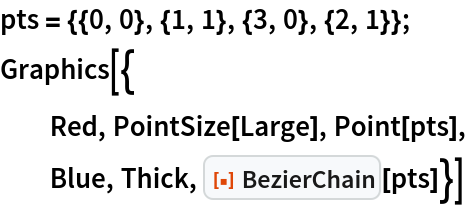Out=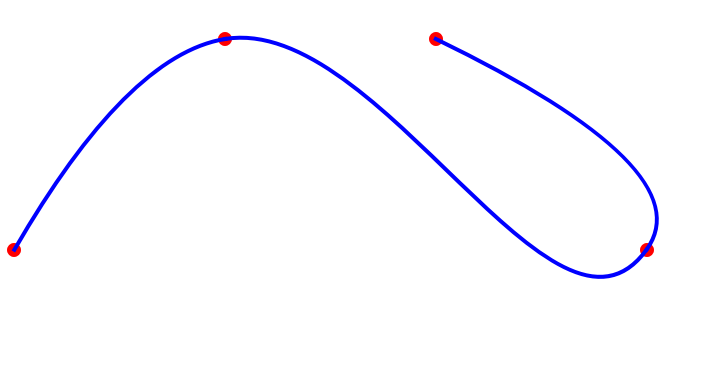In contrast, an ordinary Bézier curve will not go through all the control points:

 In:=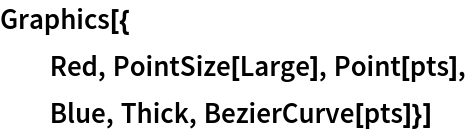Out=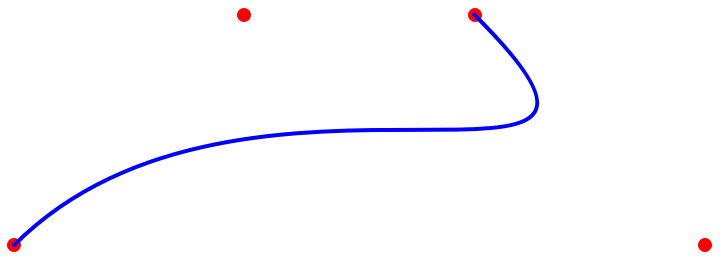### Scope (1)

Make a curve that flows through points in 3D:

 In:=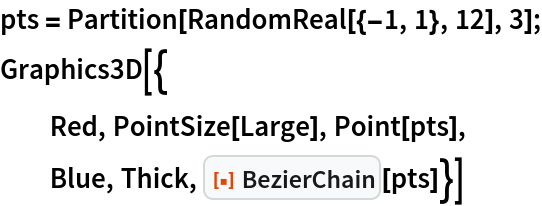Out=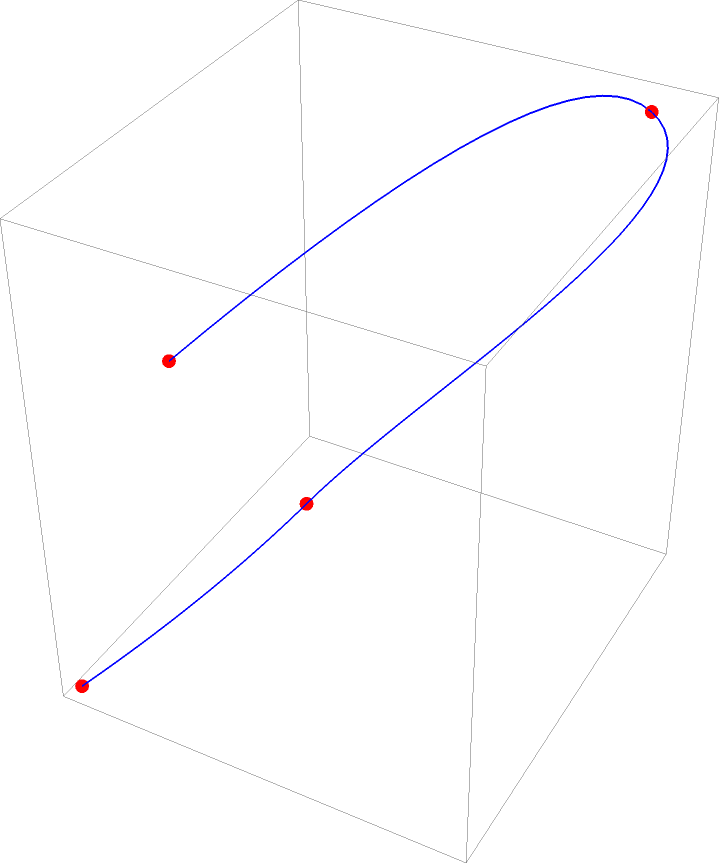### Options (4)

#### CurveType (1)

The "CurveType" option changes the type of 2D curve returned:

 In:=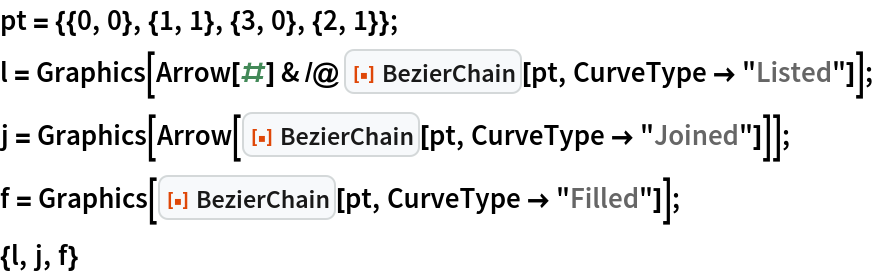Out=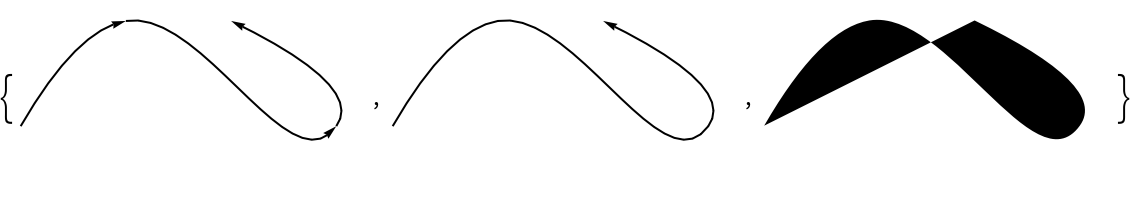#### Swing (3)

The "Swing" option, which has an effective range of 0<n≤1, controls how curvy the connection is between Bézier sections:

 In:=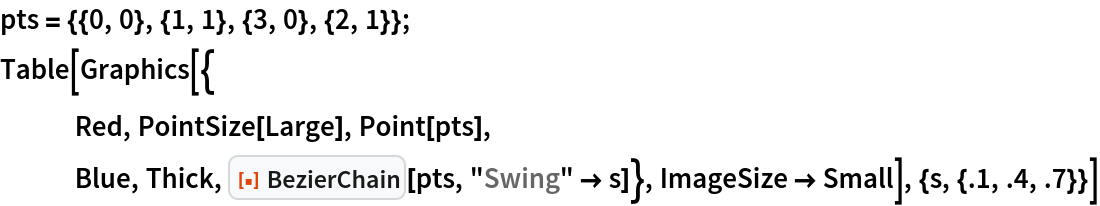Out=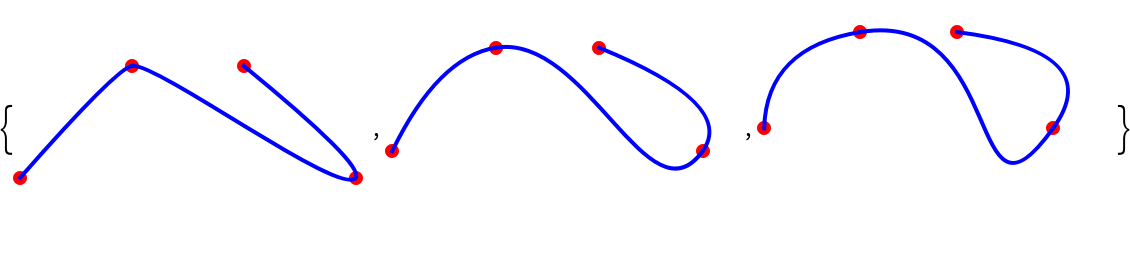A swing value of 0 is equivalent to using Line:

 In:=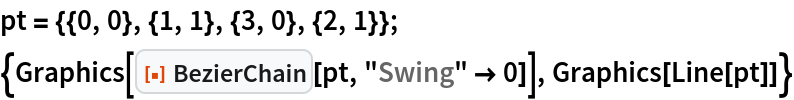Out=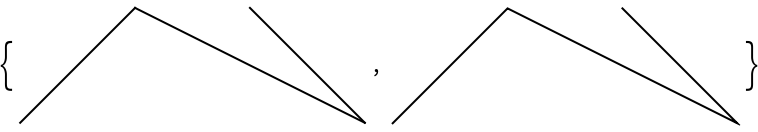In:=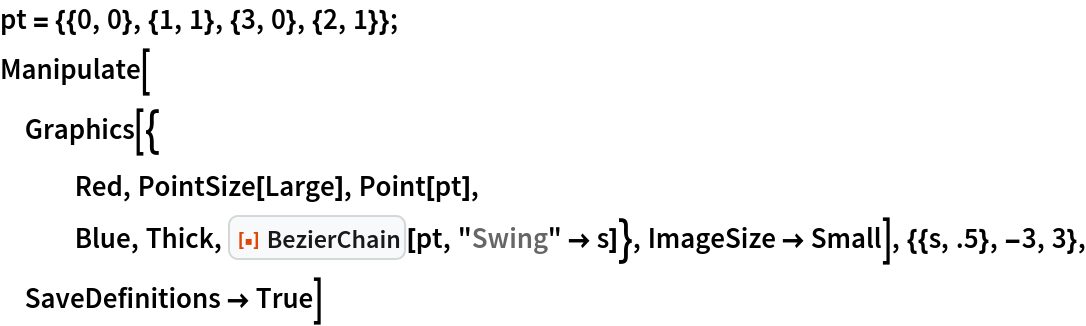Out=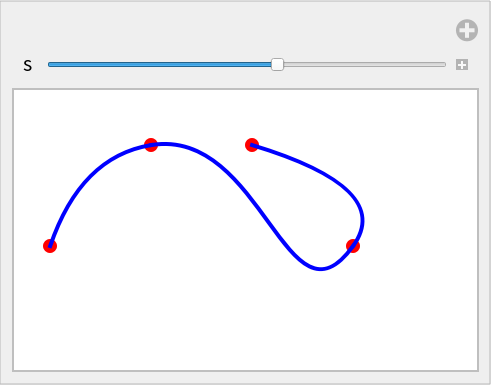### Possible Issues (1)

If the first and last points coincide (to make it a closed curve), they will not be smoothly connected, but instead will show a cusp:

 In:=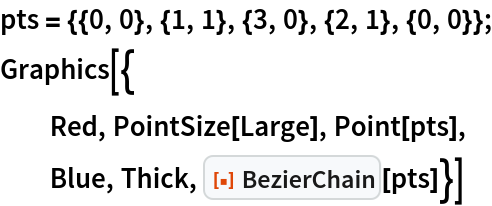Out=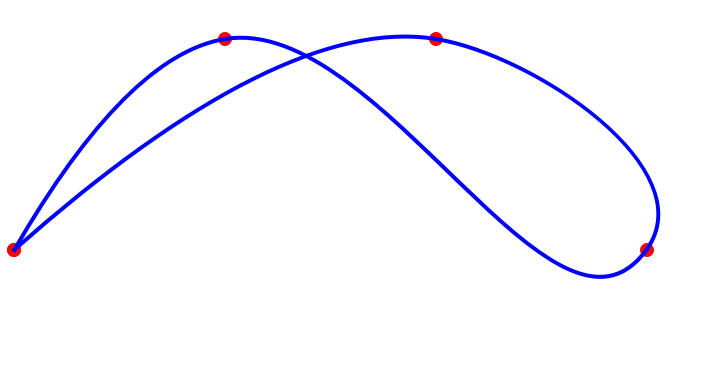### Applications (2)

Trace a path in 2D or 3D space:

 In:=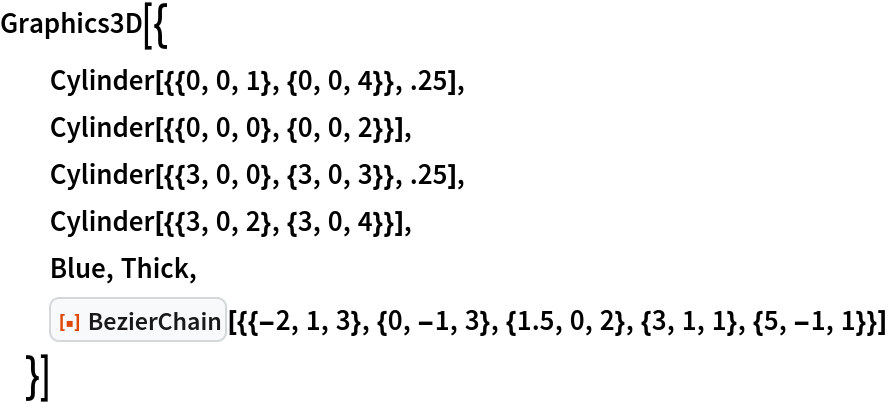Out=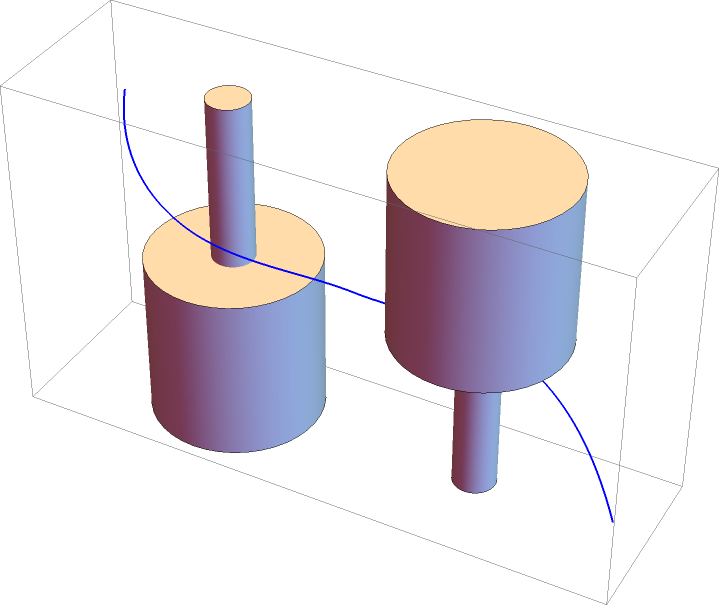Make the points dynamic, as in this Manipulate:

 In:=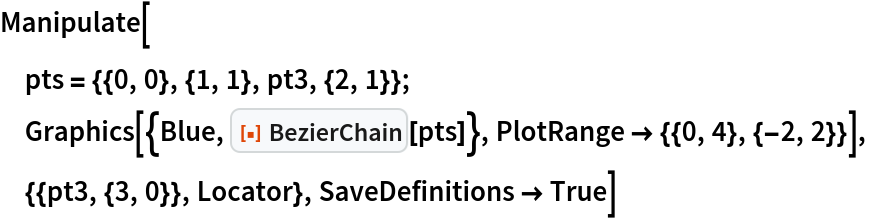Out=### Neat Examples (2)

Simulate handwriting:

 In:=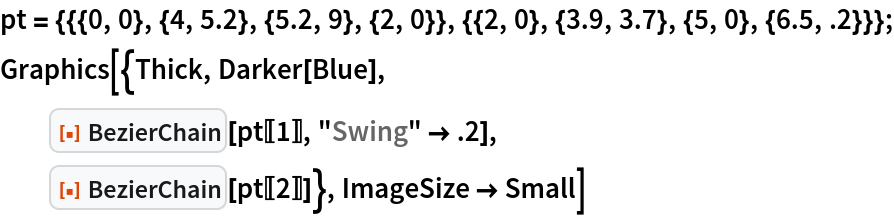Out=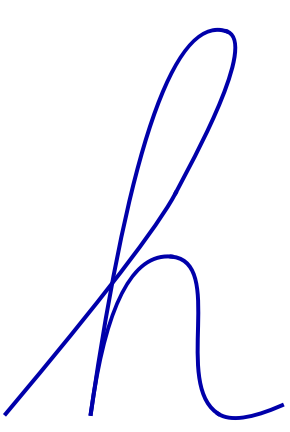Braid tubes:

 In:=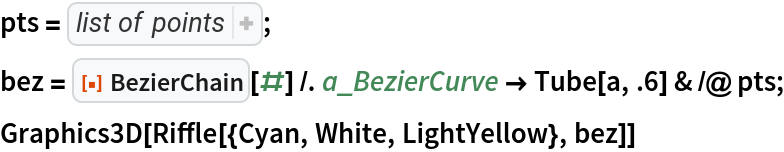Out=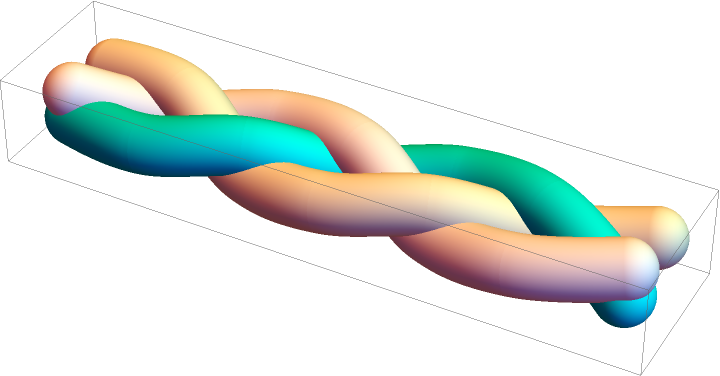Mark Greenberg

## Version History

• 1.1.0 – 10 March 2021
• 1.0.0 – 25 January 2021